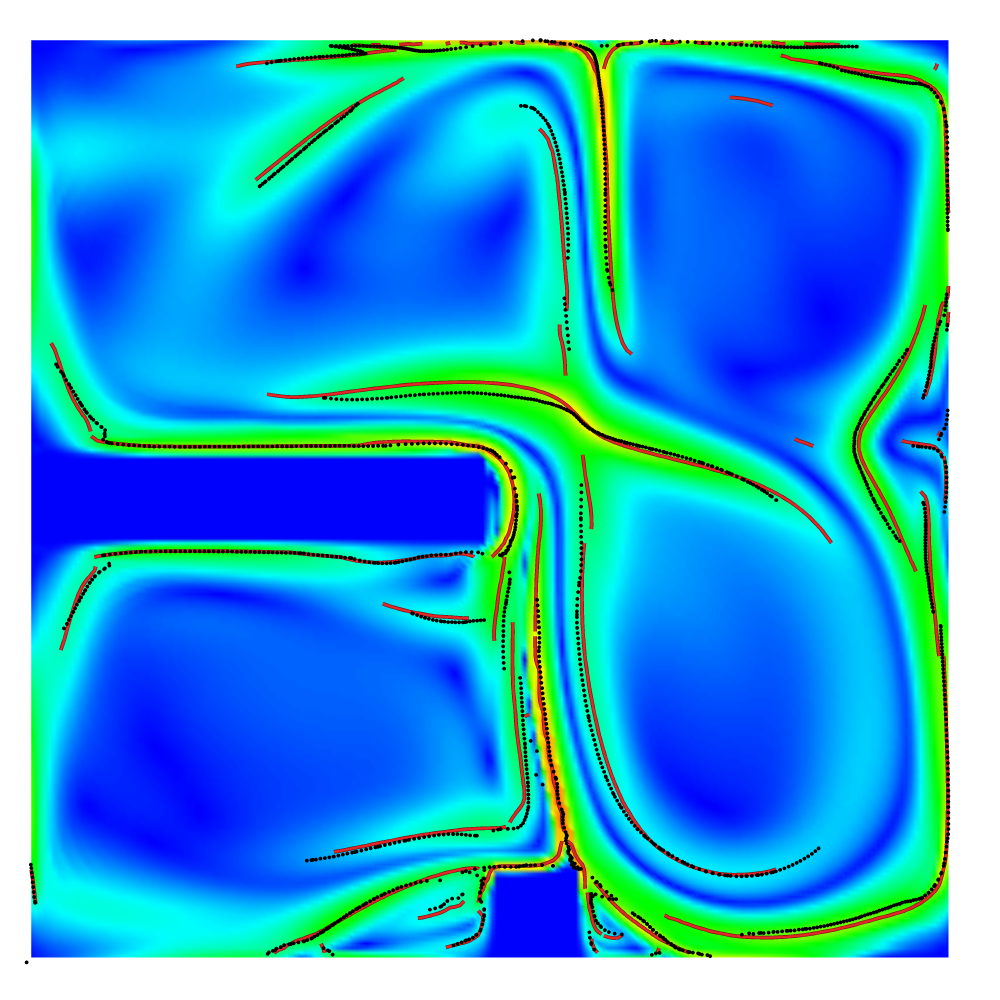F. Sadlo, M. Üffinger, T. Ertl, D. Weiskopf:

## On the Finite-Time Scope for Computing Lagrangian Coherent Structures from Lyapunov Exponents

In Topological Methods in Data Analysis and Visualization II, Springer Berlin Heidelberg, pp. 269–281, 2012.### Abstract

Lagrangian coherent structures (LCS) can be extracted from time-dependent vector fields by means of ridges in the finite-time Lyapunov exponent (FTLE). While the LCS approach has proven successful in many areas and applications for the analysis of time-dependent topology, it is to some extent still an open problem how the finite time scope is appropriately chosen. One has to be aware, however, that the introduction of this finite time scope in the Lyapunov exponent, where the time scope was originally infinite, is largely responsible for the recent success of the FTLE in analysis of real-world data. Hence, there is no general upper bound for the time scope: it depends on the application and the goal of the analysis. There is, however, a clear need for a lower bound of the time scope because the FTLE converges to the eigenvalue of the rate of strain tensor as the time scope approaches zero. Although this does not represent a problem per se, it is the loss of important properties that causes ridges in such FTLE fields to lose the LCS property. LCS are time-dependent separatrices: they separate regions of different behavior over time. Thereby they behave like material constructs, advecting with the vector field and exhibiting negligible cross flow. We present a method for investigating and determining a lower bound for the FTLE time scope at isolated points of its ridges. Our approach applies the advection property to the points where attracting and repelling LCS intersect. These points are of particular interest because they are important in typical questions of Lagrangian topology. We demonstrate our approach with examples from dynamical systems theory and computational fluid dynamics.

### Available Files

[BibTeX] [DOI] [PDF] [Slides]